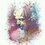# The Number Sequence and The Number Polynomial

I just got an idea of sequences that can be created out of each and every positive integer. I would like to start it with an example :

For the number 6 , the sequence would be 2,6,12,20,30,42,56 ........... it is because 6 = 1 x 6 or 6 = 2 x 3 . We could observe that the lowest gap between the factors is in the case of 2 x 3 . Hence if 2 is considered as the variable 'n' , 2 x 3 can also be written as n x (n+1) . Hence , when we substitute 1 in place of 'n' ,it is 2 , if substituted 2 the 2nd term would be 6 , 3rd term would be 12 and so on . Hence the number sequence of 6 is 2,6,12,20,30,42,56 .........

Yes , the next i would like to describe the number polynomial . Again , let me describe it with the number 6 . Hence we know that the factors of 6 are 1,2,3,6 . The polynomial of 6 would be f(x) = 1x^2 + 2x^6 + 3x^12 + 6x^42. Hence the idea is simple . The first term's coefficient would be the first divisor and the power of the term would be the 1st term of the number sequence of 6 i.e. 2 . The second term of the polynomial would be 2 as the second factor is 2 while the power of the term would be the second term in the number sequence of 6 i.e. 6 . Hence the last of the polynomial would have the coefficient as 6 , the last divisor with the power being the 6th term in the sequence of 42.

I hope you will like this idea . Thanks for reading my note and like & reshare the note if you like it !!!Note by Sriram Venkatesan
6 years, 4 months ago

This discussion board is a place to discuss our Daily Challenges and the math and science related to those challenges. Explanations are more than just a solution — they should explain the steps and thinking strategies that you used to obtain the solution. Comments should further the discussion of math and science.

When posting on Brilliant:

• Use the emojis to react to an explanation, whether you're congratulating a job well done , or just really confused .
• Ask specific questions about the challenge or the steps in somebody's explanation. Well-posed questions can add a lot to the discussion, but posting "I don't understand!" doesn't help anyone.
• Try to contribute something new to the discussion, whether it is an extension, generalization or other idea related to the challenge.

MarkdownAppears as
*italics* or _italics_ italics
**bold** or __bold__ bold
- bulleted- list
• bulleted
• list
1. numbered2. list
1. numbered
2. list
Note: you must add a full line of space before and after lists for them to show up correctly
paragraph 1paragraph 2

paragraph 1

paragraph 2

[example link](https://brilliant.org)example link
> This is a quote
This is a quote
    # I indented these lines
# 4 spaces, and now they show
# up as a code block.

print "hello world"
# I indented these lines
# 4 spaces, and now they show
# up as a code block.

print "hello world"
MathAppears as
Remember to wrap math in $$ ... $$ or $ ... $ to ensure proper formatting.
2 \times 3 $2 \times 3$
2^{34} $2^{34}$
a_{i-1} $a_{i-1}$
\frac{2}{3} $\frac{2}{3}$
\sqrt{2} $\sqrt{2}$
\sum_{i=1}^3 $\sum_{i=1}^3$
\sin \theta $\sin \theta$
\boxed{123} $\boxed{123}$